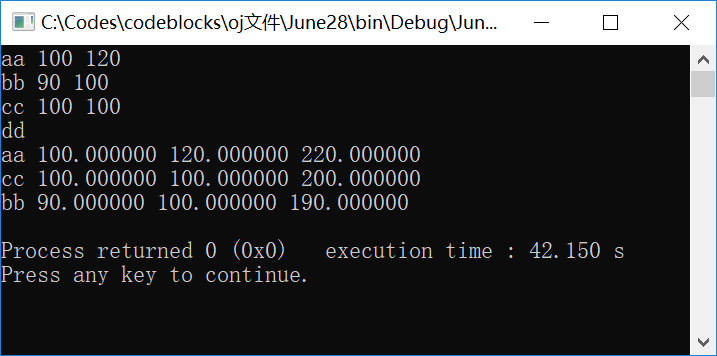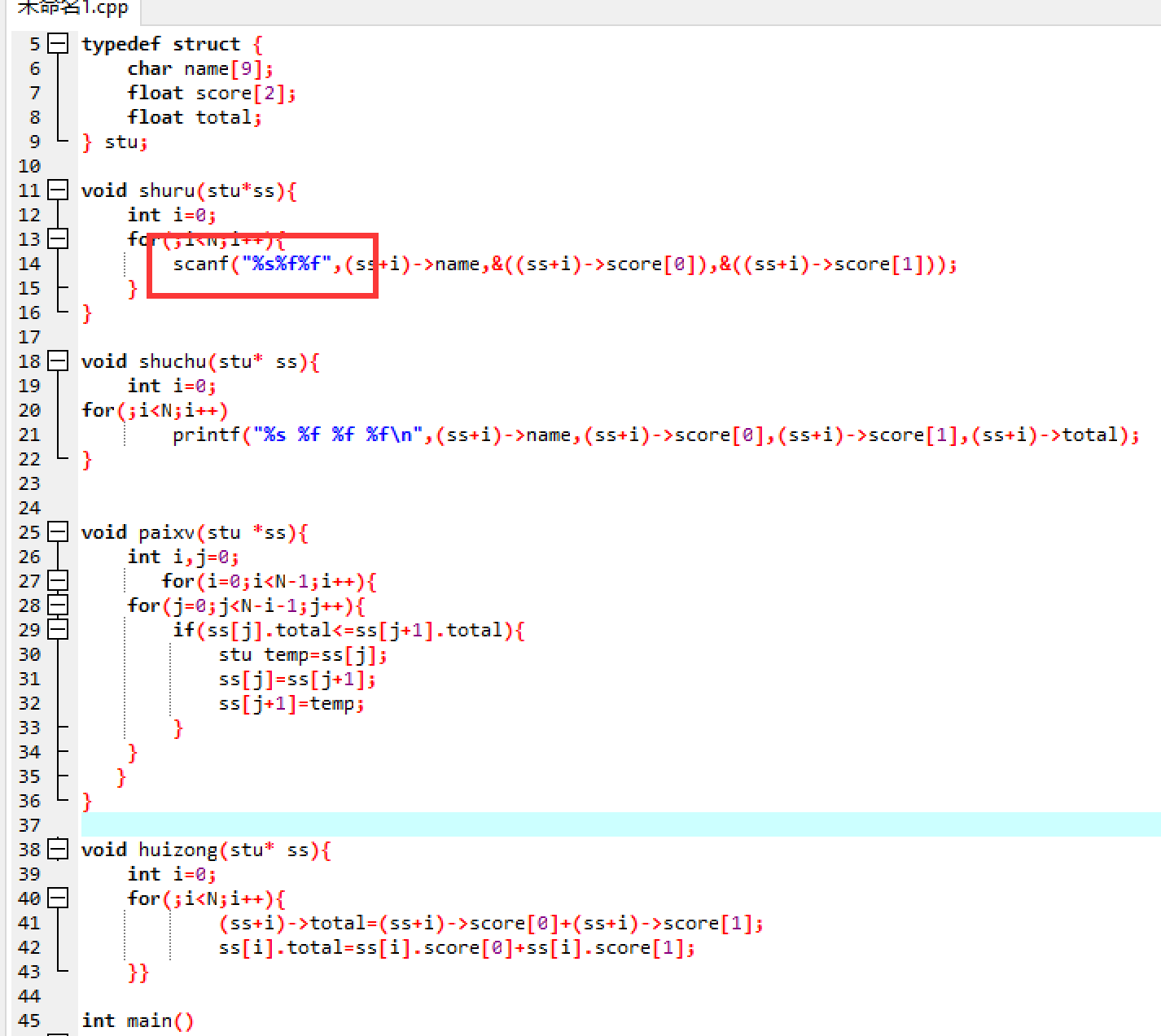C语言中结构体数组用 for循环和 scanf 输入 比应输入行数多一行

C语言中 结构体数组用 for循环和scanf输入 比应输入行数多一行，这是为什么？

3组学生信息的输入，成绩汇总，排序，打印``````#include <stdio.h>
#include <stdlib.h>
#include <string.h>
#define N 3
typedef struct {
char name;
float score;
float total;
} stu;

void shuru(stu*ss){
int i=0;
for(;i<N;i++){
scanf("%s%f%f\n",(ss+i)->name,&((ss+i)->score),&((ss+i)->score));
}
}

void shuchu(stu* ss){
int i=0;
for(;i<N;i++)
printf("%s %f %f %f\n",(ss+i)->name,(ss+i)->score,(ss+i)->score,(ss+i)->total);
}

void paixv(stu *ss){
int i,j=0;
for(i=0;i<N-1;i++){
for(j=0;j<N-i-1;j++){
if(ss[j].total<=ss[j+1].total){
stu temp=ss[j];
ss[j]=ss[j+1];
ss[j+1]=temp;
}
}
}
}

void huizong(stu* ss){
int i=0;
for(;i<N;i++){
(ss+i)->total=(ss+i)->score+(ss+i)->score;
ss[i].total=ss[i].score+ss[i].score;
}}

int main()
{
stu  ss[N];
int i,j=0;

shuru(ss);
huizong(ss);
paixv(ss);
shuchu(ss);

return 0;
}

``````

2个回答